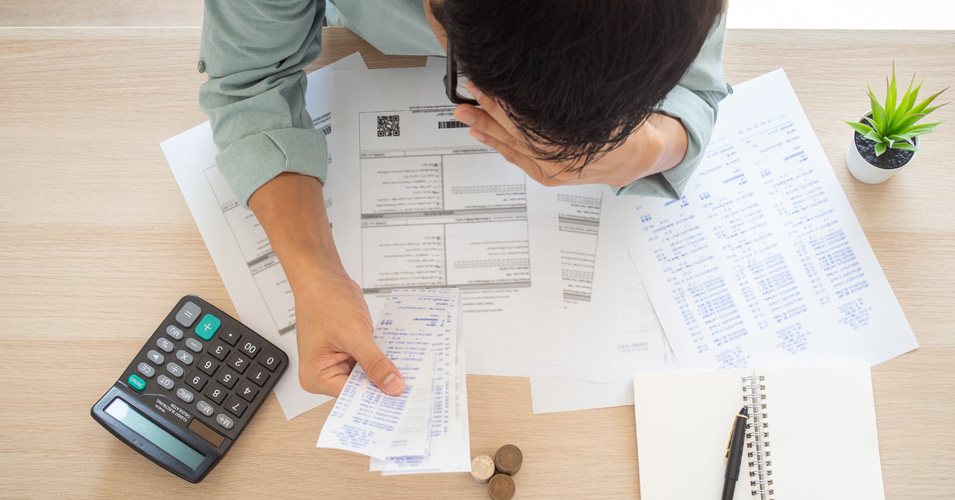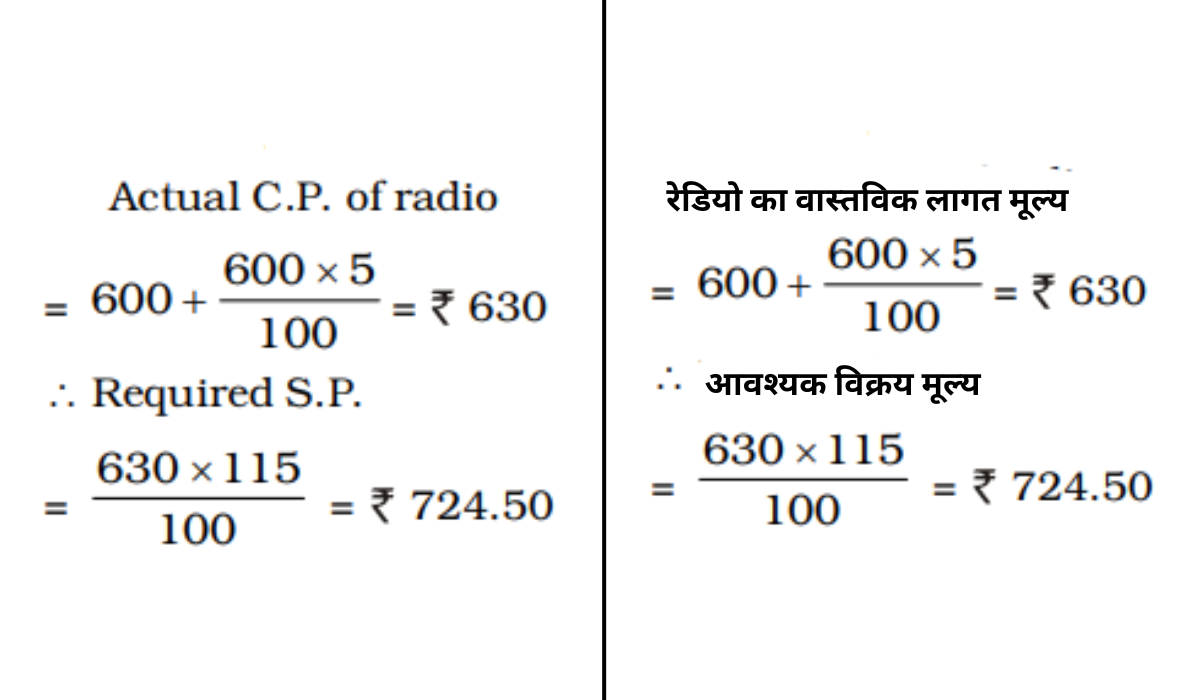• Save

# Profit and Loss Aptitude Questions for Competitive ExamsProfit and loss is an important section of the Mathematics subject. In addition to solving questions related to profit and loss, students need to have a basic knowledge of purchase price, selling price, profit and loss formulas. It is impossible to calculate the profit and loss without understanding the formulas, so to get good marks in the competitive exam, every student should practice these questions in the aptitude section.

So here, I am sharing important questions related to profit and loss for your practice. These questions will help you to improve your score by simply cover the different types of Profit and Loss Problems.

Get the Practice Test and Questions of Profit and Loss as well as Test Series for competitive exams by one click.

## Profit and Loss Aptitude Questions

Q :

The cost price of a radio is Rs. 600. The 5% of the cost price is charged towards transportation. After adding that, If net profit to be made is 15%, then the selling price of the radio must be

(A) Rs. 664.50

(B) Rs. 684.50

(C) Rs. 704.50

(D) Rs. 724.50

Explanation :Q :

A manufacturer fixes his selling price at $$33{1\over3}\%$$ over the cost of production. If the cost of production goes up by 12 % and manufacturer raises his selling price by 10%, his percentage profit is

(A) 35%

(B) $$28{3\over8}\%$$

(C) $$36{5\over9}\%$$

(D) $$30{20\over21}\%$$

Q :

A piece of land came to a person through three middlemen each gaining 20%. If the person purchased the land for Rs. 3,45,600 the original cost of the land was:

(A) Rs.1,75,800

(B) Rs.2,00,000

(C) Rs.1,00,000

(D) Rs.1,50,000

Q :

A shopkeeper sold his goods at half the list price and thus lost 20 %. If he had sold on the listed price, his gain percentage would be

(A) 20%

(B) 60%

(C) 35%

(D) 72%

Q :

The cost price of an article is Rs x. It is marked up by 200%. It is sold at Rs. 540 after giving 25% discount. What is the value of x (in Rs)?

(A) 300

(B) 240

(C) 360

(D) 250

Q :

A merchant has 1000 kg sugar, some part of which he sells at 8 % profit and the rest at 18 % profit. He gains 14 % on the whole. The quantity sold at 8 % profit is:

(A) 400 kg

(B) 560 kg

(C) 600 kg

(D) 640 kg

Q :

A trader sold an article at profit of 20%. Had he bought that article at 60% less price and sold it at Rs 90 less, then he would have gained 50%. What is the value (in Rs) of cost price?

(A) 250

(B) 300

(C) 150

(D) 200

Q :

A & B jointly made a profit of Rs. 1650 and they decided to share it such that 1/3 of A's profit is equal to 2/5 of B's profit. Then profit of B is

(A) Rs. 850

(B) Rs. 800

(C) Rs. 700

(D) Rs. 750

Q :

By what fraction selling price ( S.P. ) must be multiplied to get the cost price ( C.P. ) if the loss is 20%?

(A) $${5\over4}$$

(B) $${6\over5}$$

(C) $${4\over5}$$

(D) $${8\over5}$$

Q :

Last year Mr. A bought two paintings. This year he sold them for Rs. 20,000 each. On one, he made a 25 % profit and of the other he had a 25 % loss. Then his net profit or loss is

(A) He earned more than Rs. 2000

(B) He earned less than Rs . 2000

(C) He lost more than Rs. 2000

(D) He lost more than Rs. 2000